Academy > Technical Analysis > Exponential Moving Average (EMA): The Most Important Moving Average Indicator >

# Exponential Moving Average (EMA): The Most Important Moving Average Indicator

2022-06-28 03:08:56

## Summary:

• The Exponential Moving Average (EMA) places weight on recent price data and is used for day trading to generate buy and sell signals. It’s similar to the Weighted Moving Average (WMA).
• The EMA indicator can also be used for long-term trading by adjusting the n-period settings to 50, 100, or 200 periods; a cross between two EMAs could indicate a trend reversal.
• A trader can open a long trade once the price falls below the EMA line. Conversely, they can open a short trade once the price rises above the EMA line.

Moving averages are measurement tools used to identify the direction of a price trend. Appearing as trend lines on the candles, it indicates the average price of an asset over an n-period. The two common types of moving average indicators are exponential moving average and simple moving average.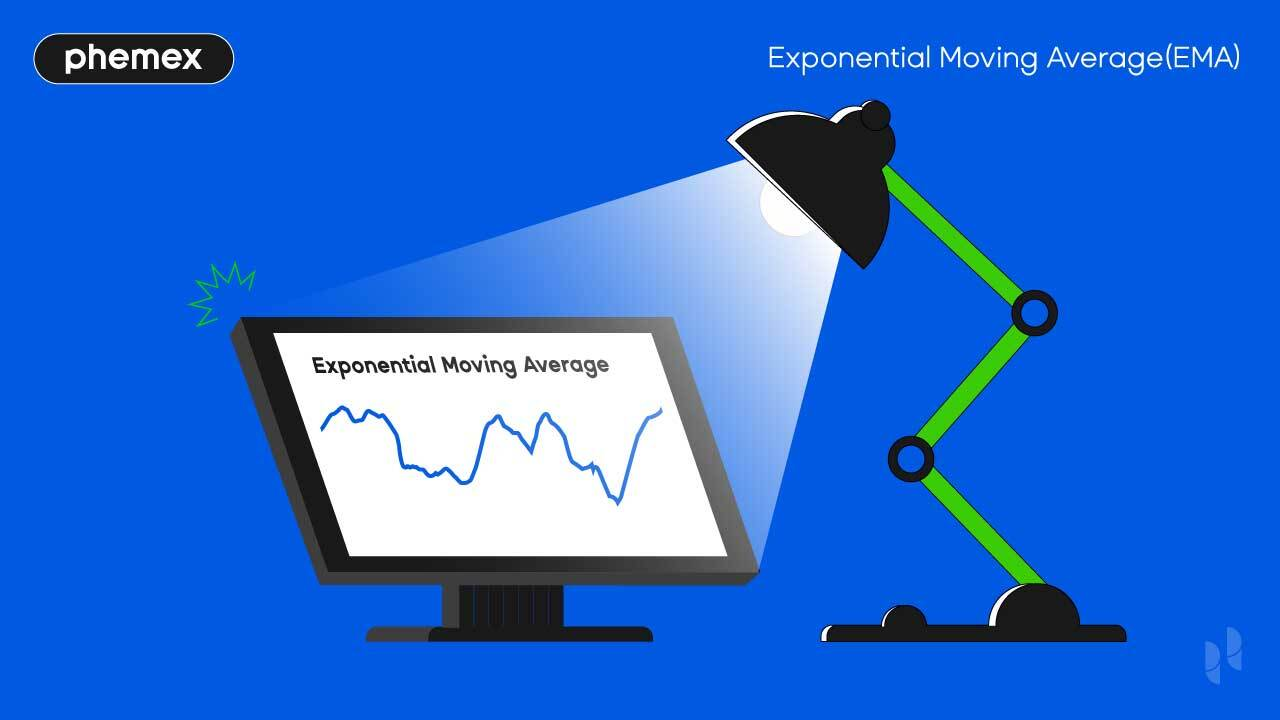## What Is The Exponential Moving Average (EMA) Indicator?

The Exponential Moving Average (EMA) is a type of moving average indicator that places weight on recent price trends, and is used for trend confirmation, divergence, and crossover trading. Along with the Simple Moving Average (SMA), the EMA is arguably the most important moving average indicator in trading, and among the top 10 most popular indicators for crypto trading.

The EMA indicator appears as a line that oscillates around the Bitcoin candles. If the price moves above the EMA, we might be in a bullish uptrend. Upon confirmation from other candles, a trader can open a long trade. If the price moves below the EMA, we’re in a bearish downtrend and a trader can consult other indicators to confirm the trend before opening a short trade. The price of Bitcoin will only temporarily deviate from a moving average before coming back to test it, and then trade above or below it.

In crossover trading, a trader can implement multiple EMAs based on different n-periods to trade cross. For example, a 20-day EMA and a 50-day EMA crossing could indicate a reversal on the current trend. The formula is based on daily closing averages and smoothening to filter historic price data for the current trend.## Who Invented The Exponential Moving Average?

The Exponential Moving Average has existed since 1963, making it one of the oldest trading indicators. Notable contributions to the study of exponential smoothening were made by Robert Goodell and Charles Holt.

Robert Goodell was a mathematician and a writer who specialized in exponential smoothening. His book series, Smoothening, Forecasting, And Prediction of Discrete Time, released in 1963, was used as the foundation for the EMA indicator.

Charles Colt, a university professor at the Business School of the University of Texas in Austin, also contributed to the area of exponential smoothening.

The EMA indicator is most commonly used for stocks and commodities trading, but has since been adopted by crypto traders. Prior to the introduction of digital trading software, traders manually calculated the EMA.

## How to Calculate the Exponential Moving Average

The Exponential Moving Average is initially based on a regular moving average (MA). The MA is easier to calculate because it’s the sum of average closing prices without any weighted data. The EMA indicator uses previous EMA averages and gradually decreases the value of historic data.

Here is the formula for the EMA:

The variables for “today” and “yesterday” refer to Bitcoin’s current price averages. The “a” stands for smoothening or the amount of weight we choose to place on the equation – this is often 2. Exponential Moving Average calculation always depends on the previous results.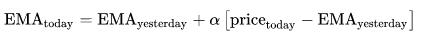The default EMA n-period on Phemex is 9 which means it calculates prices over 9 days on the chart.

The weight on moving average exponential values decreases the further they deviate from the current average. Placing weight on recent prices makes the EMA more sensitive than indicators such as the SMA. The line moves faster, providing day trading signals as they occur.

## How To Use Exponential Moving Average?

Let’s try out the EMA on the Phemex platform.

1 Head to the “Markets” and pick from hundreds of trading pairs; in this example, we’ll use the BTC/USDT chart. Start by opening a pair and click on “Indicators” at the top: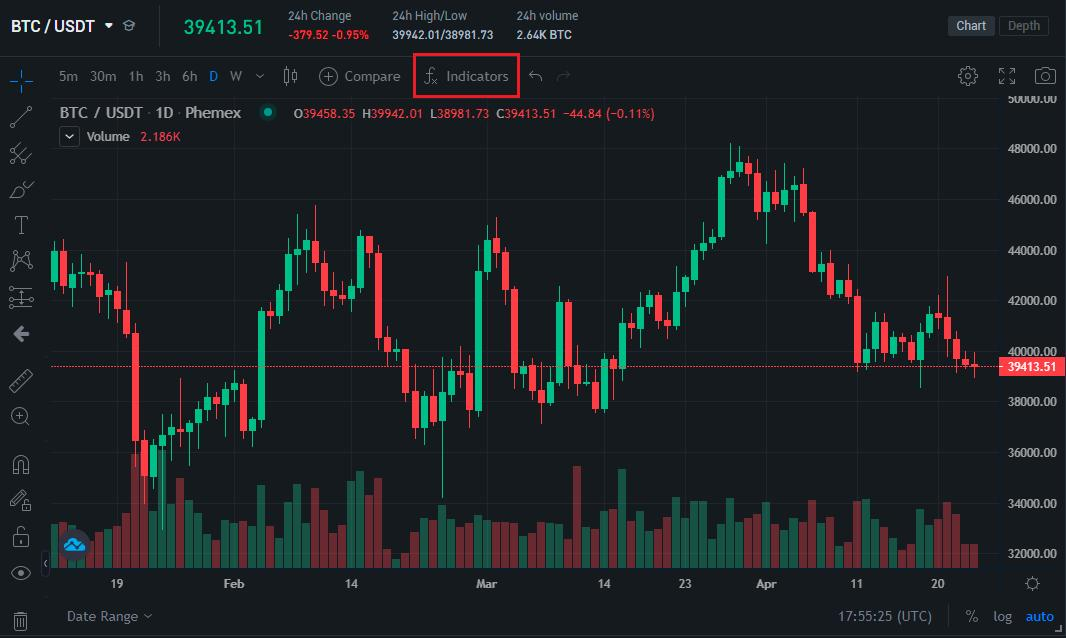2 The indicator dropdown will prompt a search bar where you can input “Moving Average Exponential”: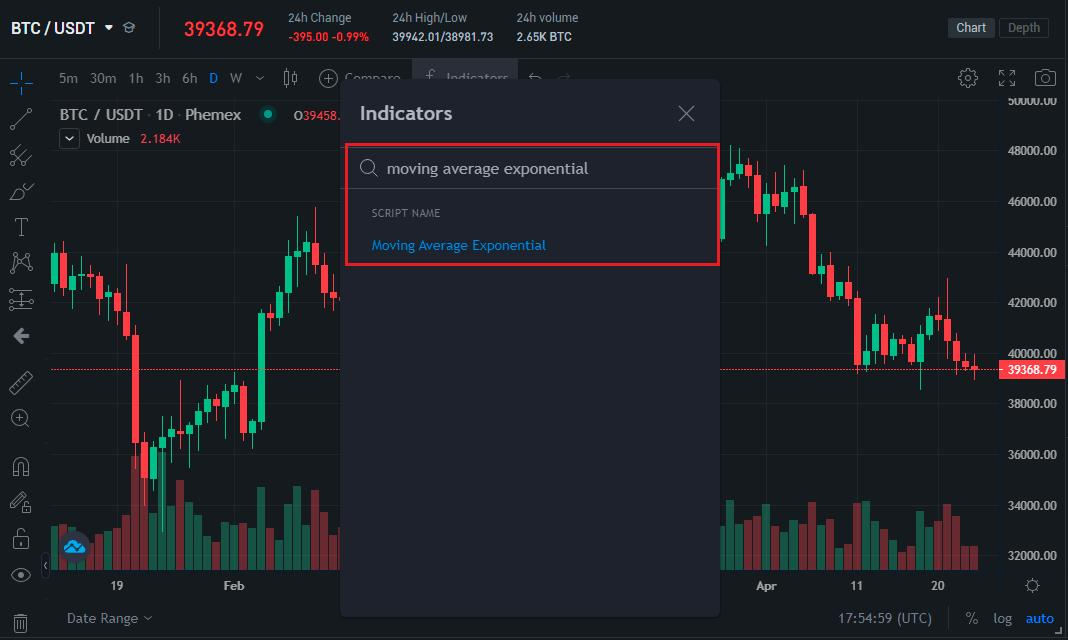3 Left-click on the indicator to activate it on the Bitcoin chart: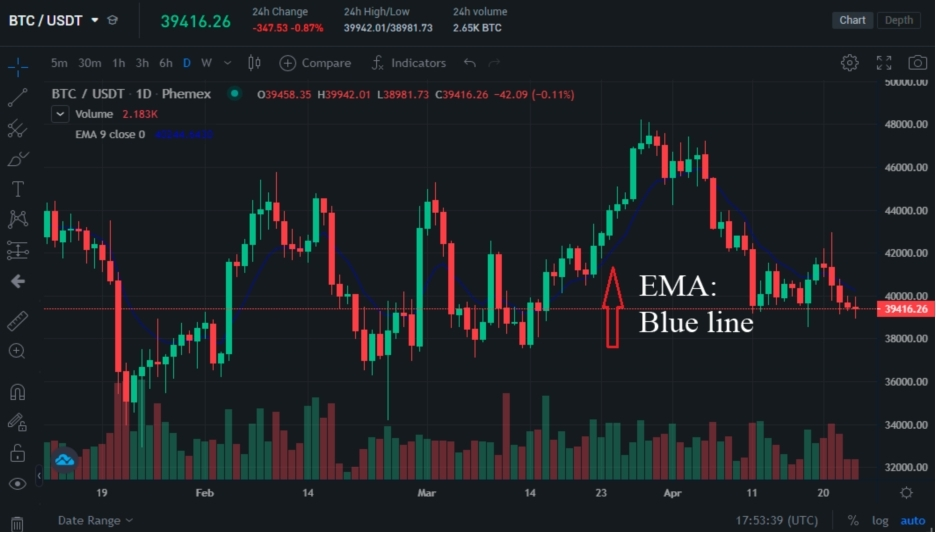4 The EMA will appear as a blue line oscillating above and below the Bitcoin candles. We can see that the EMA line moves closely to the candles due to high sensitivity – signaling support and resistance levels for the volatile crypto markets. Support levels are arbitrary price floors where the price is likely to reverse to the upside. Resistance levels are arbitrary price ceilings where the price is likely to reverse to the downside.

## What Is A Double or Triple Exponential Moving Average?

Traders can activate dual or triple EMAs for crossover trading. Exponential moving averages can be adjusted for an n-period and output different values. A trader could simultaneously activate a 9-EMA, 20-EMA, 50-EMA, 100-EMA, 200-EMA, etc.

Click on “Indicators” again and activate more EMAs. Once you’ve activated them, press on “Settings” to adjust the timeframe for each: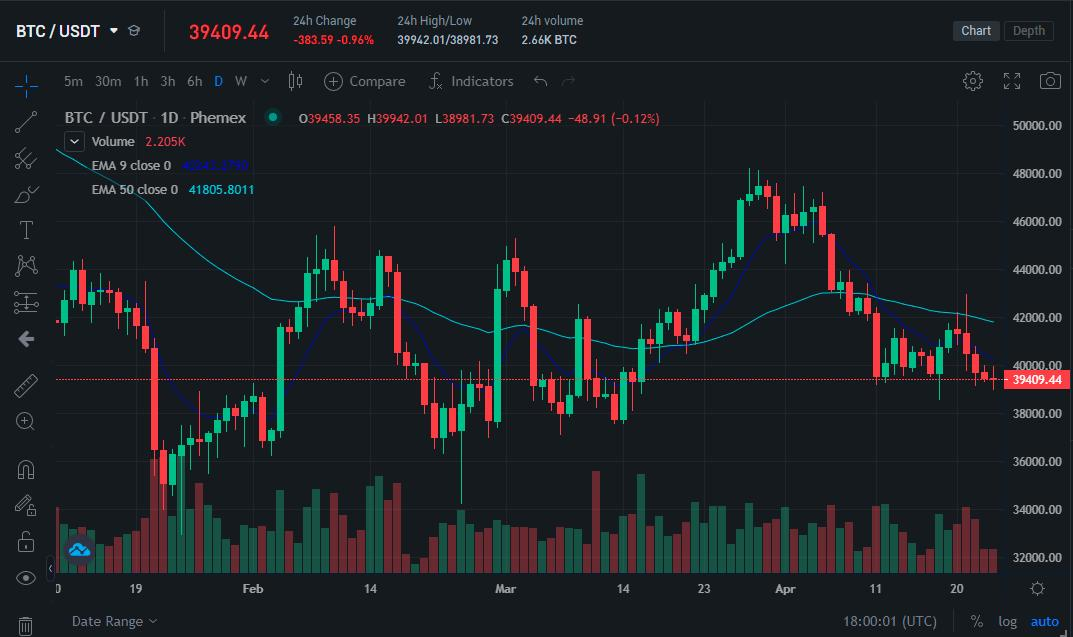For this example, we want to use the 9-EMA and 50-EMA. To convert the 9-period EMA to a 50-period EMA, we only have to extend the length to 50. We can load them simultaneously: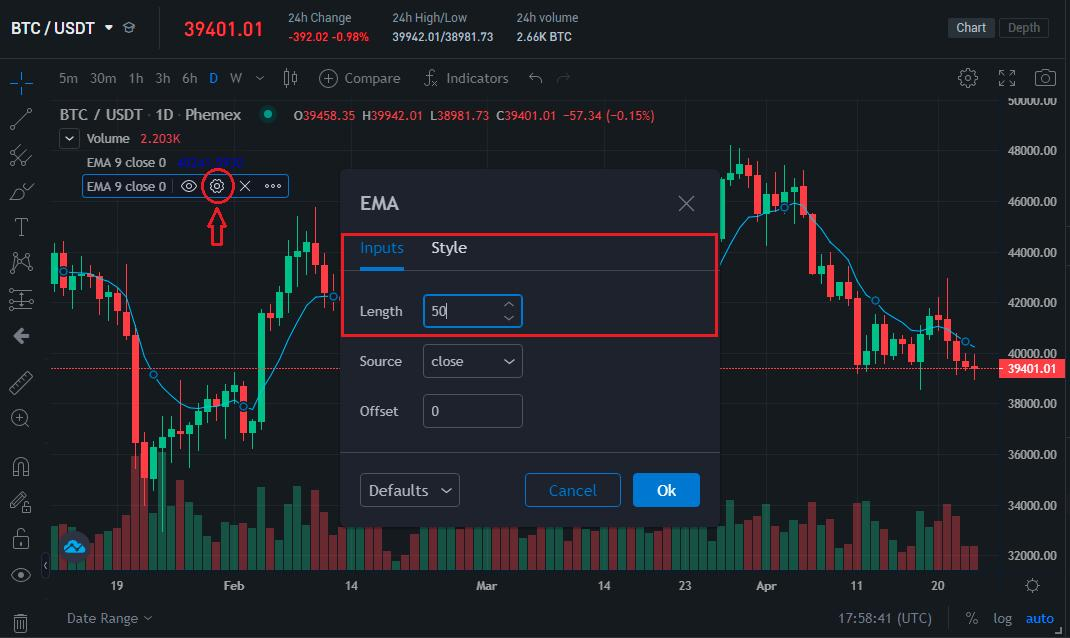We now have a double EMA setup. The light blue line is the 50-period EMA and the blue line is the 9-period EMA. We can see the shorter EMA is more sensitive and conforms to the candles because it’s based on recent averages, while the 50-period uses additional historical data.

Pro Tip: If you’re wondering which exponential moving average to use, choose long EMAs (>50-period) for value investing and short EMAs (20-period<) for short-term day trading.

## How To Trade With The Exponential Moving Average?

The Exponential Moving Average can be used fortrend trading, crossover, and divergence. The EMA is functional as a standalone indicator, but it’s recommended to combine it with other indicators such as the Relative Strength Index (RSI) or Stochastic RSI (StochRSI).The moving average exponential indicator is used in crossover trading to send buy and sell signals. Multiple EMAs are required to trigger a signal. For example, a 9-period EMA and a 50-period EMA could be used to send buy and sell signals. Using the 1D (daily) chart we can test the effectiveness of this strategy: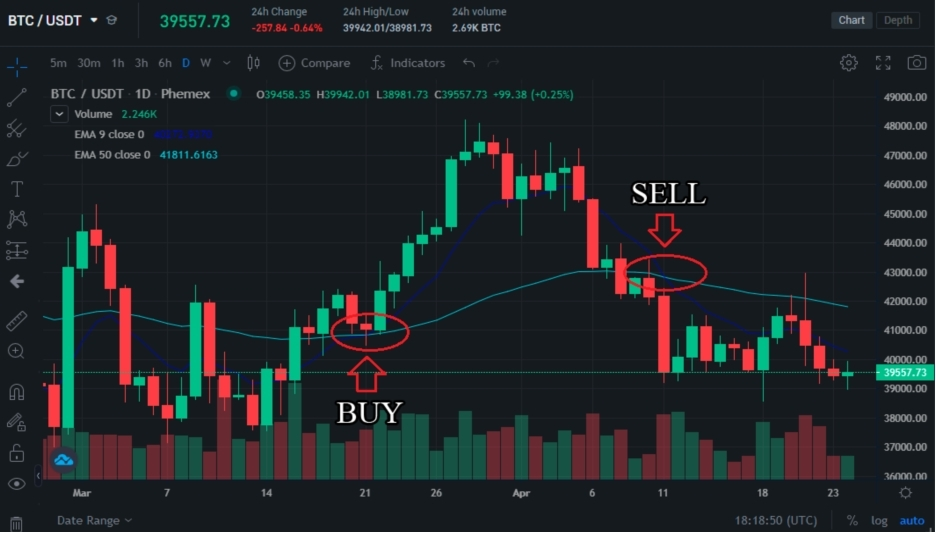If the dark blue line (9-EMA) crosses the light blue line (50-EMA) and goes above it, this is a bullish signal and we can open a short order. If the light blue line crosses the dark blue line and goes above it, this is a bearish signal and we can open a short order.

Once the two lines crossed, Bitcoin went on a 15% upward rally from \$40,000 up to \$48,000 and sustained the trend for a couple of days. Once the EMA decreased, the indicator picked up on the bearish divergence and sent a sell signal.

Day trading with the EMA should be done using short-term charts such as 4H (hourly) and adjusted for shorter n-periods. Avoid using the indicator in isolation and use trend oscillators such as the Relative Strength Index (RSI) that indicate “Overbought” and “Oversold” levels.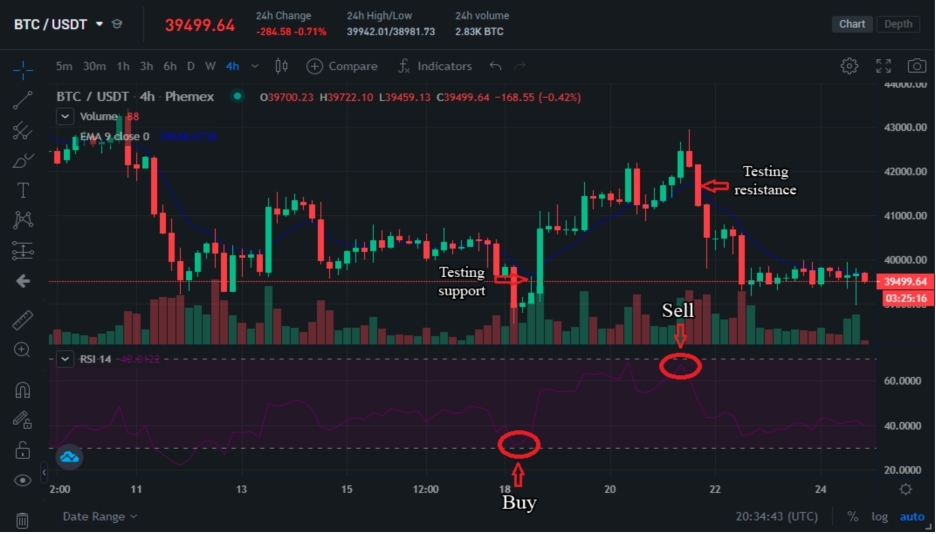The RSI indicator and the EMA are merged for day trading.

Ideal trading opportunities arise when the RSI oscillator reaches extreme overbought or oversold levels. We can see that the price decreased below the EMA which presents a great long opportunity and this is matched with an oversold value on the RSI indicator. The price then rose above the EMA indicator, matched with an overbought value.

In the former example, the price rose from \$39,000 to \$43,000 and in the latter example the price fell from \$43,000 to \$39,000. Simply put: Long when the price falls below the EMA and short when the price rises above the EMA. Ensure the signal is matched with extreme readouts from the RSI oscillator.

## Simple Moving Average vs Exponential Moving Average: Which Is Better?

The Simple Moving Average and Exponential Moving Average are similar indicators. The SMA places equal weight on all historic values. The EMA places weight on the latest averages and uses smoothening to gradually decrease the importance of historic data in relation to the current trend. The two indicators can be activated simultaneously: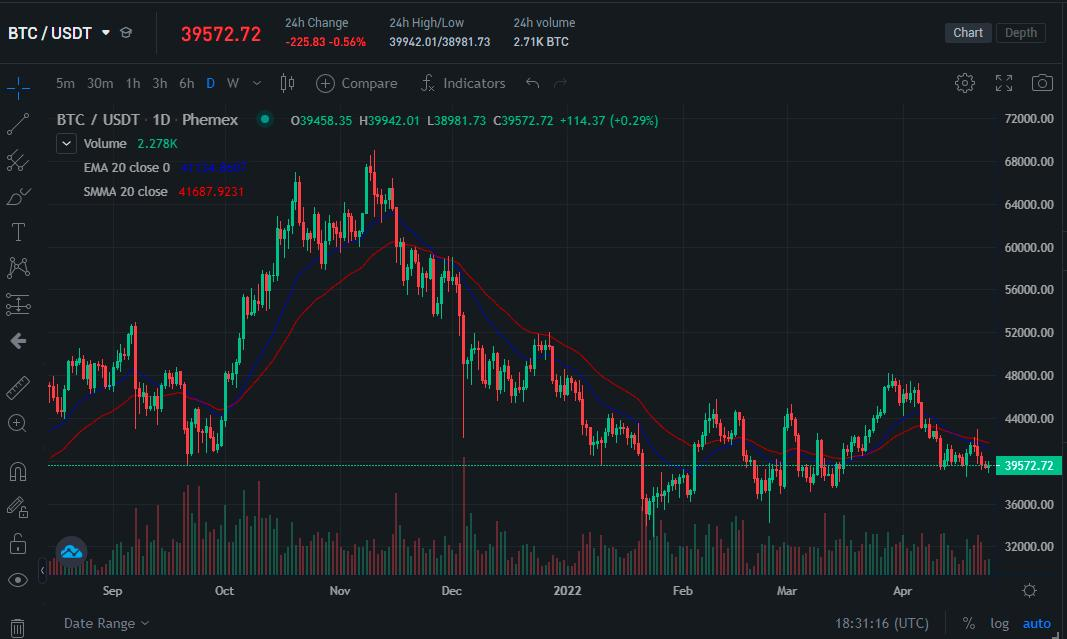Simple Moving Average vs Exponential Moving Average.

The EMA is more sensitive compared to the SMA and oscillates more with the candles. We can notice how the EMA (blue) sticks to the Bitcoin candles and moves close to the current trend, whereas the SMA is slightly separated. The SMA is recommended for long-term investing while the EMA is recommended for day trading.

The EMA is also different compared to the regular moving average (MA) indicator. The MA indicator only calculates the averages for an n-period without smoothening applied: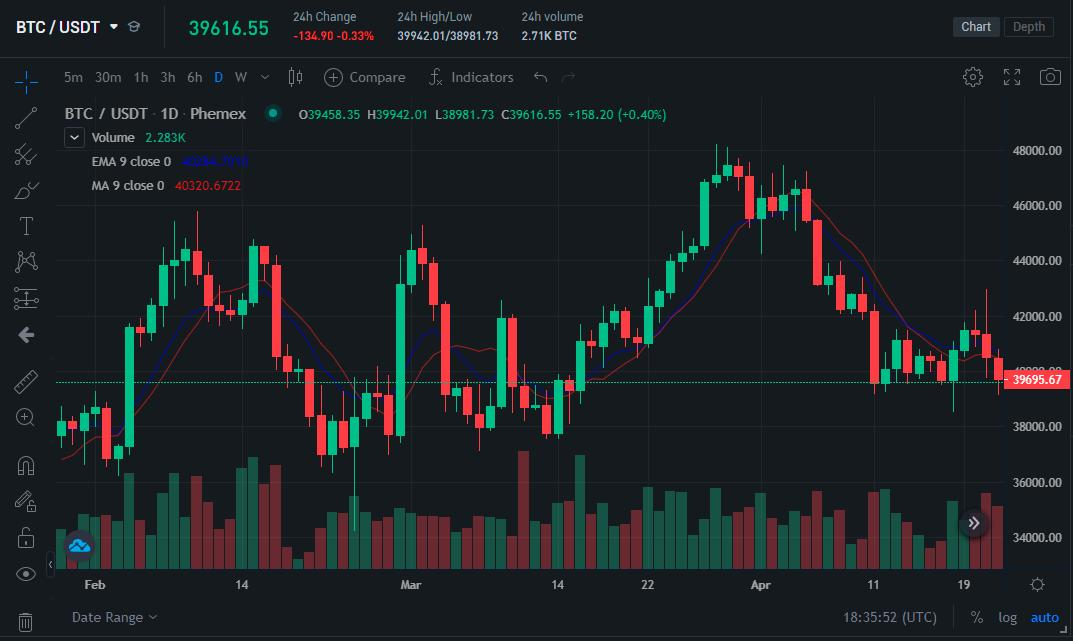Moving Average vs Exponential Moving Average.

The MA indicator (red) and the EMA indicator (blue) have similar values on the Bitcoin chart; however the MA indicator is further separated from the Bitcoin candles than the EMA indicator which indicates the EMA is more sensitive.

## Conclusion

Moving average indicators have existed for 100+ years and most indicators are based on a moving average. For example, the MACD indicator is based on a double EMA. The most effective EMA trading strategy is to implement a double EMA for crossover trading. Buy signals are generated when a less-sensitive (longer) EMA crosses a more-sensitive (shorter) EMA and rises above it.

The Exponential Moving Average is most similar to the Weighted Moving Average as both indicators place an emphasis on recent price data. The Simple Moving Average can output near-identical values if the n-periods are the same on both indicators.

Phemex | Break Through, Break FreeRegister to get \$180 Welcome Bonus!
Email